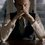# An amazing pattern!

1. Take any number $m$ (let's say 3).

2. Write all the numbers starting from $1$ in one line.

3. Eliminate numbers at the index $k$ ($k \equiv 0 \pmod{m}$)

4. Now write for each index, partial sum of the numbers till that index.

5. Eliminate numbers at the index $k$ ($k \equiv m-1 \pmod{m}$).

6. Now write for each index, partial sum of the numbers till that index.

$\vdots$

$\quad$2m-1. Eliminate numbers at the index $k$ ($k \equiv 2 \pmod{m}$).

$\quad$2m. Now write for each index, partial sum of the numbers till that index.

For example, for $m=3$,

$\begin{array}{ c c c c c c c c c c c c c} 1 & 2 & 3 & 4 & 5 & 6 & 7 & 8 & 9 & 10 & 11 & 12 & 13\\ 1 & 2 & & 4 & 5 & & 7 & 8 & & 10 & 11 & & 13\\ 1 & 3 & & 7 & 12 & & 19 & 27 & & 37 & 48 & & 61\\ 1 & & & 7 & & & 19 & & & 37 & & & 61\\ 1 & & & 8 & & & 27 & & & 64 & & & 125\\ \end{array}$

$\displaystyle \text{We get a sequence of} \ n^m \text{!}$

Prove that this happens for any number of numbers and for any natural number $m$.

PS - This is not a challenge. This is seeking help.Note by Kartik Sharma
3 years, 12 months ago

This discussion board is a place to discuss our Daily Challenges and the math and science related to those challenges. Explanations are more than just a solution — they should explain the steps and thinking strategies that you used to obtain the solution. Comments should further the discussion of math and science.

When posting on Brilliant:

• Use the emojis to react to an explanation, whether you're congratulating a job well done , or just really confused .
• Ask specific questions about the challenge or the steps in somebody's explanation. Well-posed questions can add a lot to the discussion, but posting "I don't understand!" doesn't help anyone.
• Try to contribute something new to the discussion, whether it is an extension, generalization or other idea related to the challenge.

MarkdownAppears as
*italics* or _italics_ italics
**bold** or __bold__ bold
- bulleted- list
• bulleted
• list
1. numbered2. list
1. numbered
2. list
Note: you must add a full line of space before and after lists for them to show up correctly
paragraph 1paragraph 2

paragraph 1

paragraph 2

[example link](https://brilliant.org)example link
> This is a quote
This is a quote
    # I indented these lines
# 4 spaces, and now they show
# up as a code block.

print "hello world"
# I indented these lines
# 4 spaces, and now they show
# up as a code block.

print "hello world"
MathAppears as
Remember to wrap math in $$ ... $$ or $ ... $ to ensure proper formatting.
2 \times 3 $2 \times 3$
2^{34} $2^{34}$
a_{i-1} $a_{i-1}$
\frac{2}{3} $\frac{2}{3}$
\sqrt{2} $\sqrt{2}$
\sum_{i=1}^3 $\sum_{i=1}^3$
\sin \theta $\sin \theta$
\boxed{123} $\boxed{123}$

Sort by:

(1) I have fixed your LaTeX. The correct code here is "\begin{array}".

(2) This result is known as Moessner's Theorem.

- 3 years, 12 months ago

Oh I see. Thanks! I will look into the references. BTW, can you give a simplified proof?

- 3 years, 12 months ago

- 3 years, 12 months ago

I'm afraid I don't know the proof. I think it's a hard result.

- 3 years, 12 months ago

Yeah. I am not able to find an understandable proof yet. And its generalizations to the way Conway has done in his book, is magical indeed.

- 3 years, 12 months ago

@Mark Hennings @Pi Han Goh @Ishan Singh Please also fix the latex!

- 3 years, 12 months ago

This is seriously magic. Another math-e-magical thing with which you can fascinate a primary school student and can "irritate" a mathematician.

(Another such thing is the Goldbach's Theorem).

- 3 years, 12 months ago